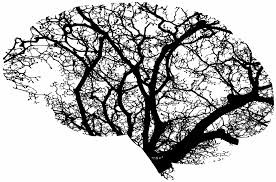## HOW TO CATEGORIZE YOUR DEEP LEARNING PROJECT ?

Hypothetically anything in this world can be solved and design through deep learning / machine learning. All those problems which is solved through human can be solved through machine learning because as human , machine is also learning through data, But at this stage we don't have that much advancement in this technology due to which we cannot achieve that goal. In machine learning (DL) recently problems are divided into three main problems type and combinations of it. We mainly have classification, Regression and sequence to sequence learning type problems in machine learning (DL). We will discuss all of this three type of problems in details.

For any deep learning problem we need to first figure out in which type of problem that machine learning (DL) problem fits in because architecture is highly dependent on type of problem. If you able to find out your problem fits in which of the above categories .It will be easier for you to deal with that problem .

## CLASSIFICATION

This type of problem we mainly found in supervised learning where we have labelled data. Problem of deep learning where we have to find classes of data given than we assume that type of problem as classification problem. Let say we have 1000 images of dog and cat. We want to classify each images of image in dog or cat category than we will generate model through deep learning . This type of problem we could find in Vision, Audio, Tabular, Text (NLP) . we have to train our model to classify input falls in which of these classes.

In classification problems we generally use Softmax activation function after last layer to get probabilities of each classes. We output same number of output as classes which we want make classification as in our dog vs cat . We will output two number in which each one predict probabilities of dog and cat.

In classification problem type we generally use cross entropy as LOSS function to improve our model. In our dog vs cat we would have chosen categorical - cross-entropy as a loss function to improve our model.

## REGRESSION

This type of problem is also used in supervised learning problem. In this type of problem we need to predict one or multiple continuous variable number which is in FLOAT. Let say we want to predict sales for future by using companies past data. Then here we will train our model based on all data of transaction as input and our companies pattern of business and can predict future sales through that pattern. Regression problem in mainly found in tabular data. Any Where we want find continuous variable number , we can used methods and tricks which we used in regression. Regression type of problem can be also found in Vision, Audio, Text. In vision we mainly found this problem in bounding boxes problem.

In regression problem we generally use simple linear layer at the end with no activation function so that we can get accurate values. Secondly we always train our model by taking log of dependent variable /Y variable so that model predicts small values. To get actual values we need to take exponentials of that value which we obtain our output is equals to number of dependent variable. In the above case which is one in regression problem we mainly [mean square error ] loss function to improve our model.

## SEQUENCE TO SEQUENCE PROBLEM

This type of problem is found when we have variable length input and variable length output and we need to design model like this. This model take variable length input and gives variable length output, like we see in machine translation where input is some language words and machine will output some other language sentences. If we provide text as " I LOVE YOU " machine will translate to its French sentence " JE T'AIME" in machine translation from English to French. You can find Sequence to sequence problem type in many domains like Audio to text conversation in speech recognize system. This is also used in audio to audio machine translation.

The main idea to solve this problem is to first encode your input to some representation and then pass that encoded representation to neural networks and then decode that representation to other output representation for training purpose you need large labelled data corpose.

## CONCLUSION

In this blog we have seen different types of deep learning problem which a machine learning engineer face in its life span, and also gives some of the solution which community adopt when they see such type of problem